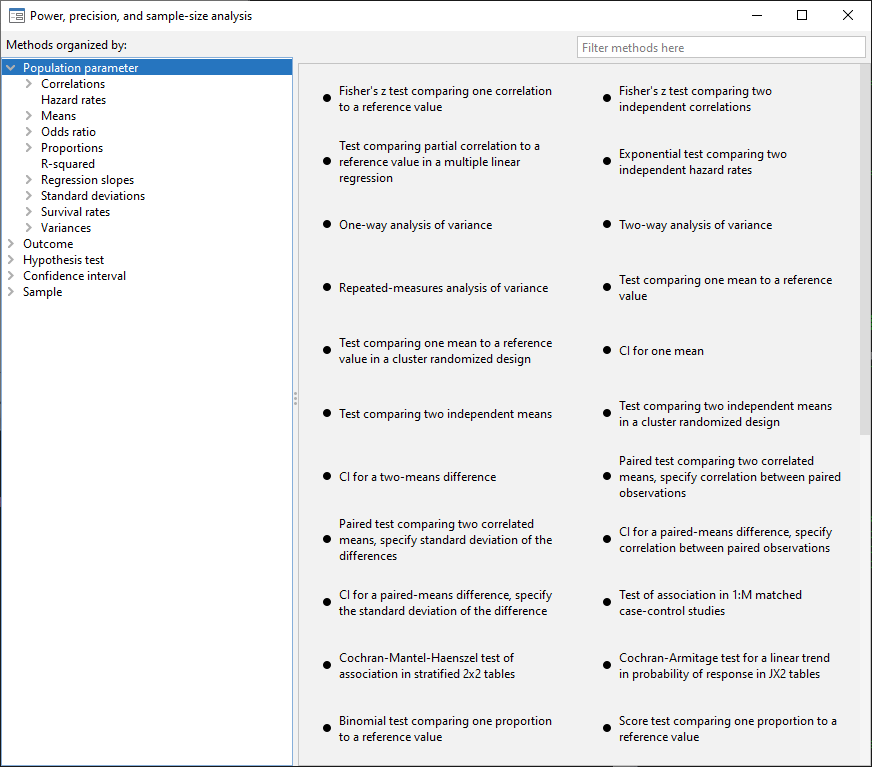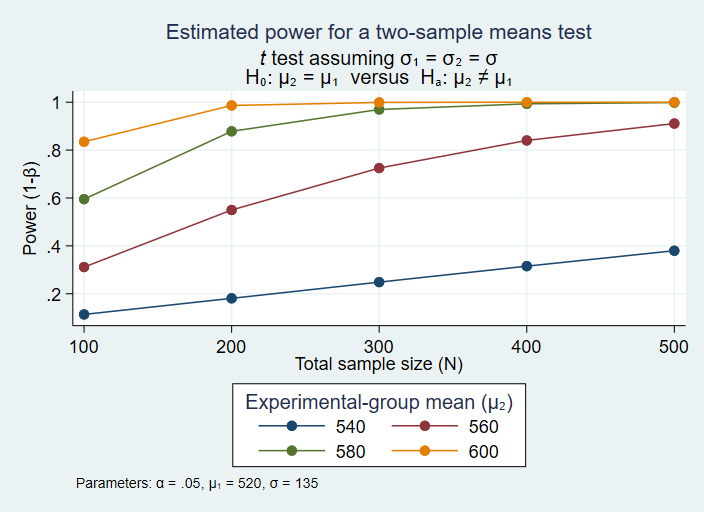Classic comparisons

Means (t-tests)

Proportions

Variances

Correlations

McNemar’s test

Clustered data

ANOVA

One-way models

Two-way models

Repeated-measures models

Linear regression

Slope test

Coefficient-of-determination test

Partial-correlation test

Contingency tables

Cochran—Mantel—Haenszel test

Matched case—control studies

Cochran—Armitage trend test

Cluster randomized designs

One-sample mean

Two-sample means

One-sample proportion

Two-sample proportions

Log-rank test

Survival analysis

Log-rank test of survival functions

Cox proportional hazards model

Exponential regression

Clustered Data

Solve for

Power

Sample size

Number of clusters

Cluster size

Effect size (minimum detectable effect)

Designs

Matched case–control studies

Cohort studies

Cross-sectional studies

Balanced

Unbalanced

Specify lists of

Alpha values

Power levels

Beta values

Sample sizes

Ratios of sample sizes

And more

Multiple scenarios using

All combinations of values

Parallel lists of valuesControl panel interface

Select analysis type from descriptive summaries

Specify list of power, sample size, or other levels

Automated tables and graphs

Customize tables and graphs

Command interface too (of course)

Tables

Automated

Customized

Graphs

Automated

Customized

Simple or comparative

Save results to dataset